# SBI PO Pre Quantitative Aptitude Quiz- 20

## SBI PO Pre Quantitative Aptitude Quiz 2022

Quantitative Aptitude is the most significant part of almost all competitive exams. Candidates appear to be having difficulty with Quantitative Aptitude Questions, so they need to practice more. Even if the portion is difficult, if you practice enough questions and learn the basic concepts, you can surely do well. In this article, we have come up with the SBI PO Pre Quantitative Aptitude Quiz to enhance your preparation. This SBI PO Pre Quantitative Aptitude Quiz will assist you to cope with the tough competition. This SBI PO Pre Quantitative Aptitude Quiz is available to you at no cost. Through this SBI PO Pre Quantitative Aptitude Quiz, you will learn formulas and short tricks to solve the Quantitative Aptitude Questions. Candidates must solve this SBI PO Pre Quantitative Aptitude Quiz to improve their exam preparation.

Directions (1-5): The given table show the S.I. and C.I. rate per annum given by five lenders.

 Lenders S.I. rate C.I. rate A 12% 15% B – 20% C 10% – D – 20% E 25% –

Note: Some values are missing, you must calculate these values according to question.

1. Lender A, lends some amount at S.I. for 3 years and another amount, which is 1500 more than the previous one, at C.I. for 2 years. Each sum earns equal amount. Find the amount lends at C.I.
(a) 12900
(b) 13500
(c) 15000
(d) 14400
(e) 11500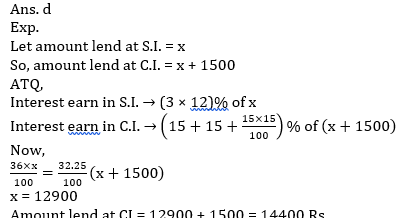2. Lender C lends a sum for 2 years at S.I. then after 2 year he re-lend the total amount for 2 years at C.I. and earn a total profit which is 45.2% of sum invest by him in S.I. Find the rate of C.I.
(a) 10%
(b) 11%
(c) 12%
(d) 20%
(e) 15%3. If D lend money in S.I. for 8 year he earns double Interest as he earns on same money in 2 years at C.I. then find the interest he earns on 1500 at S.I. in 3 year.
(a) 500 Rs.
(b) 360 Rs.
(c) 900 Rs.
(d) 495 Rs.
(e) 600 Rs.4. Interest earn by E in 2 years at C.I. is 25% more than the interest earns by him in 4 years at S.I. on same amount. What is the ratio of amount occurred when same sum is lend by D and E for 3 years at C.I.
(a) 36 : 125
(b) 64 : 113
(c) 64 : 125
(d) 75 : 113
(e) 216 : 125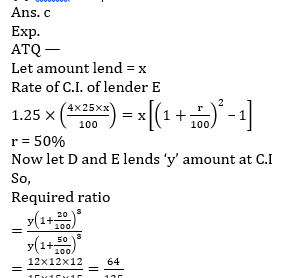5. When B lends same sum on S.I. and C.I. each, he earn interest in ratio of 3 : 2 in which he lend amount at S.I. for 3 years and at C.I. for 2 years. If difference between total interest earn in S.I. and C.I. is 2640 Rs. then find rate at S.I. lends by B ?
(a) 20%
(b) 22%
(c) 24%
(d) 10%
(e) 25%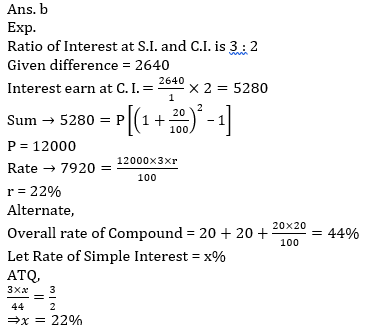Directions (6-10): In each of these questions, two equations (I) and (II) are given. You have to solve both the equations and give answer.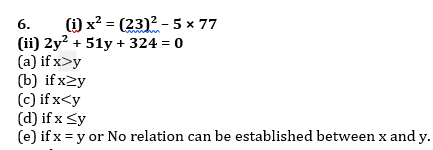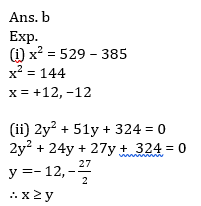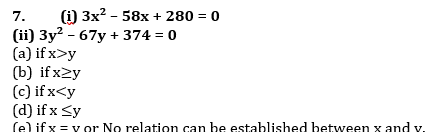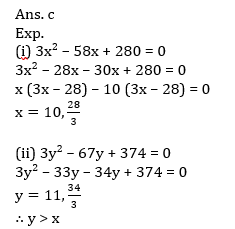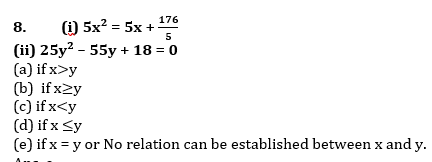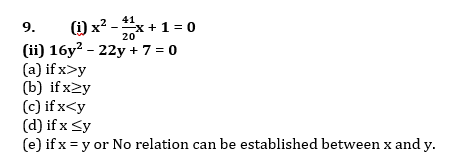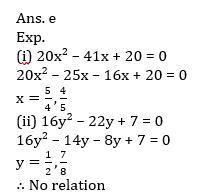Recommended PDF’s for:

### 2022 Preparation Kit PDF

#### Most important PDF’s for Bank, SSC, Railway and Other Government Exam : Download PDF Now

AATMA-NIRBHAR Series- Static GK/Awareness Practice Ebook PDF Get PDF here
The Banking Awareness 500 MCQs E-book| Bilingual (Hindi + English) Get PDF here
AATMA-NIRBHAR Series- Banking Awareness Practice Ebook PDF Get PDF here
Computer Awareness Capsule 2.O Get PDF here
AATMA-NIRBHAR Series Quantitative Aptitude Topic-Wise PDF 2020 Get PDF here
Memory Based Puzzle E-book | 2016-19 Exams Covered Get PDF here
Caselet Data Interpretation 200 Questions Get PDF here
Puzzle & Seating Arrangement E-Book for BANK PO MAINS (Vol-1) Get PDF here
ARITHMETIC DATA INTERPRETATION 2.O E-book Get PDF here
3